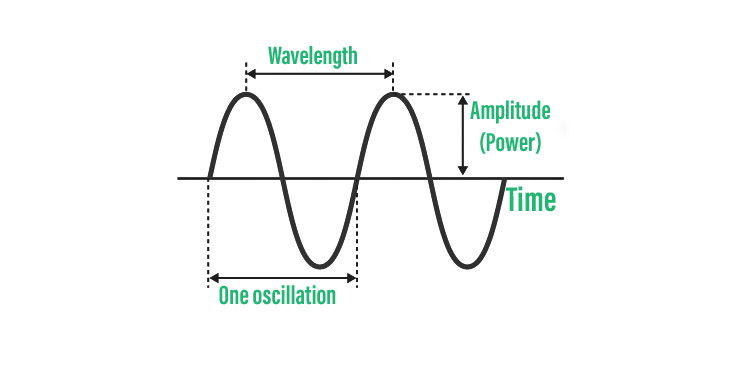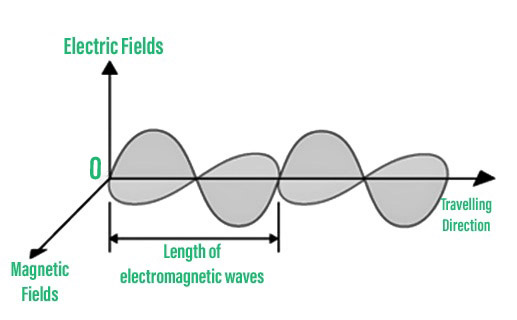Introduction to Waves – Definition, Types, Properties

• Last Updated : 05 Aug, 2021

A wave is a propagating dynamic disturbance (change from equilibrium) of one or more quantities in physics, mathematics, and related subjects, commonly described by a wave equation. At least two field quantities in the wave medium are involved in physical waves. Periodic waves occur when variables oscillate periodically around an equilibrium (resting) value at a specific frequency. A traveling wave occurs when the entire waveform moves in one direction; a standing wave occurs when two superimposed periodic waves move in opposite directions. The amplitude of vibration in a standing wave features nulls at some points when the wave amplitude seems reduced or even zero.

Wave

A wave is a disturbance in a medium that transports energy without causing net particle movement. Elastic deformation, pressure variations, electric or magnetic intensity, electric potential, or temperature variations are all examples.Pictorial representation of waves.

Characteristics of Waves

Waves include the following characteristics:

• The particles of the medium traversed by a wave vibrate only slightly about their mean positions, but they are not permanently displaced in the wave’s propagation direction.
• Along with or perpendicular to the wave’s line of travel, each succeeding particle of the medium performs a motion quite identical to its predecessors.
• During wave motion, only energy is transferred, but not a piece of the medium.

Types of Waves

The several forms of waves are listed here:

1. Transverse Waves:

Waves in which the medium moves at an angle to the wave’s direction.

Examples of transverse waves:

• Water waves (ripples of gravity waves, not sound through water)
• Light waves
• S-wave earthquake waves
• Stringed instruments
• Torsion wave

A crest is the highest point of a transverse wave. It’s a trough at the bottom.

2. Longitudinal Wave:

The movement of the particles in the medium in a longitudinal wave is in the same dimension as the wave’s movement direction.

Examples of longitudinal waves:

• Sound waves
• P-type earthquake waves
• Compression wave

Parts of longitudinal waves:

1. Compression-The particles are close together in this case.
2. Rarefaction-Where the particles are dispersed

3. Electromagnetic Waves:

These are waves that are produced and propagated without the use of a material medium, i.e., they can pass through vacuum and any other material medium.

Examples of electromagnetic waves:

• visible light
• ultra-violet light
• microwavesElectromagnetic waves.

4. Mechanical waves:

Only a material medium can produce or propagate mechanical waves. Newton’s equations of motion apply to these waves.

Examples of mechanical waves:

• waves on water surface
• waves on strings
• sound waves

Mechanical waves are of two types:

1. Transverse wave motion- The particles of the medium vibrate at right angles to the wave’s propagation direction in transverse waves. Transverse waves include string waves, surface water waves, and electromagnetic waves. The disturbance that travels in electromagnetic waves (which include light waves) is caused by the oscillation of electric and magnetic fields at right angles to the wave’s travel direction.
2. Longitudinal wave motion- Particles in the medium vibrate back and forth around their mean location along the energy propagation direction in these sorts of waves. They’re also known as pressure waves. Longitudinal mechanical waves are what sound waves are.

5. Matter waves:

These waves are linked to the movement of matter particles.

Examples of matter waves:

• electrons
• protons
• neutrons

Formula for Speed of Wave

It’s the entire distance a wave travels in a particular amount of time. The formula for calculating wave speed is as follows:

Wave Speed = Distance Covered/Time taken

Properties of Waves

The following are the primary characteristics of waves:

• Amplitude – A wave is a form of energy transmission. The amplitude of a wave is its height, which is commonly measured in meters. It is proportional to the quantity of energy transported by a wave.
• Wavelength – A wavelength is a distance between identical locations in adjacent cycles of crests of a wave. In addition, it is measured in meters.
• Period – A wave’s period is the amount of time it takes a particle on a medium to complete one complete vibrational cycle. Because the period is a unit of time, it is measured in seconds or minutes.
• Frequency – The number of waves passing a spot in a certain amount of time is referred to as the frequency of a wave. The hertz (Hz) unit of frequency measures one wave every second.

The frequency’s reciprocal is the period, and vice versa.

Period=1 / Frequency

OR

Frequency = 1 / Period

• Speed – The speed of an object refers to how quickly it moves and is usually stated as the distance traveled divided by the time it takes to travel. The distance traveled by a specific point on the wave (crest) in a given amount of time is referred to as the wave’s speed. A wave’s speed is thus measured in meters per second or m/s.

Sample Problems

Problem 1: In a specific medium, a wave travels at 900 meters per second. Calculate the wavelength of a specific point in the medium if 3000 waves pass through it in 2 minutes.

Solution:

The speed of a wave in medium v = 900 ms-1

Freq. of wave = no. of waves passing per sec (n) = 3000 waves/2 min = 3000 / 2 × 60 = 25 s

Wave length (λ) = ?

v = n × (λ)

λ = v/n

= 900/25

= 36 m

Problem 2: What distinguishes the roar of our national animal from that of a mosquito?

Solution:

The buzzing of a mosquito creates a sound of high pitch and low intensity or loudness, but the roaring of a national animal (tiger) produces a sound of low pitch and high intensity or loudness.

Problem 3: Is it feasible to tell when a vessel maintained beneath the faucet is going to overflow?

Solution:

The length of an air column is inversely related to the frequency of the note it produces. The length of the air column above the vessel reduces as the level of water in the vessel rises. It generates a sound with a decreasing frequency, i.e. the sound gets shorter. It is possible to determine whether the vessel is filled with water based on the shrillness of the sound.

Problem 4: The bottom of a ship in the sea shoots SONAR waves straight down into the saltwater. After 3.5 s, the signal reflects off the deep bottom bedrock and returns to the ship. When the ship reaches 100 km, it transmits another signal, which is received after 2 s. Calculate the depth of the sea in each example, as well as the height difference between the two.

Solution:

Velocity of SONAR waves in water C = 1500 ms-1

Time taken by be wave after reflection from the bottom of sea

2t = 3.5s

t =1.75s

Distance covered (d) = ?

C = d/t => d = c.t = 1500 × 1.75 – 2625 m

After moving 100km

The time taken by the wave = 2t = 2s

T  = 2/2 = 1s

d =?

d = 1500 × l

= 1500

The different between these two heights = 2625 – 1500

= 1125m

Problem 5: Why does a tone sound louder in an empty room than in a room with furniture and other objects?

Solution:

Sound is an energy form. The majority of the energy is absorbed by the furniture that acts as an obstruction. As a result, the strength of sound decreases, yet in an empty room, the intensity of sound remains relatively constant due to the absence of impediments, and we perceive it as louder.

My Personal Notes arrow_drop_up